Science, Maths & Technology

### Become an OU studentGeometry

Start this free course now. Just create an account and sign in. Enrol and complete the course for a free statement of participation or digital badge if available.

# Try some yourself

## Question 1

Find the area of a circle of (a) radius 8 cm, and (b) radius 15 m.

• (a)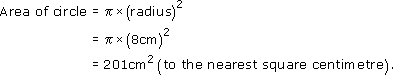• (b)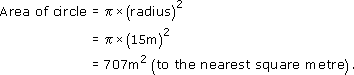## Question 2

Calculate the areas of the following shapes:

• (a) Area = 10 m × 6 m = 60 m2.

• (b) Area =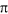(1.1 m)2 ≈ 3.80 m2.

• (c) Area =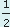× 10m × 5m = 25m2.

## Question 3

Use your answers to the previous question to find the area of turf needed for the proposed lawn shown below, which has a circular flowerbed in the middle. Round your answer to the nearest square metre.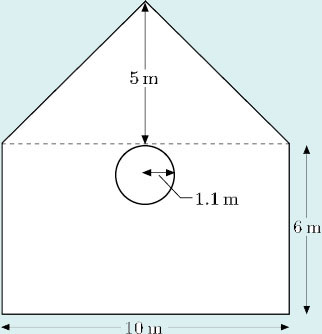Add together the areas of the rectangle and the triangle from Question 2, and subtract the area of the circle to find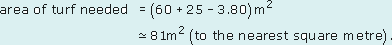## Question 4

A manufacturer produces a patio kit consisting of 28 paving slabs which are shaped so that they fit together to form rings, as shown. The outside edge of each ring is a circle, and all three circles have the same centre. Circles with the same centre are called concentric circles. The slabs are of three sizes, one for each ring of the patio. All of the slabs in a particular ring are identical.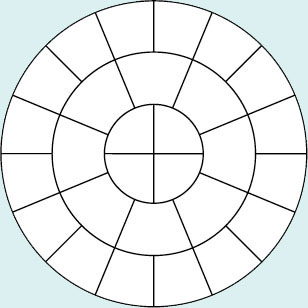The radii of the three circles are 0.4 m, 0.8 m and 1.2 m.

Making appropriate assumptions, calculate which of the three types of slab is the heaviest and which the lightest.

In the following calculation, full calculator accuracy numbers are indicated by three dots. For example the full calculator accuracy value foris written as 3.141 ... .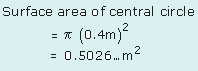There are four slabs in this circle, so each slab will have a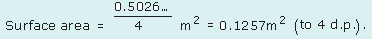The surface area of the eight slabs in the inner ring is calculated by subtracting the central circle from the circle with radius 0.8 m: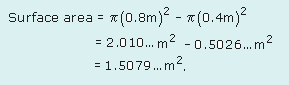There are eight slabs in the inner ring, so each slab will have a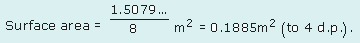Use a similar method to find the surface area of the outer ring of slabs: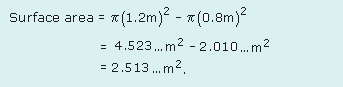There are sixteen slabs in the inner ring, so each slab will have a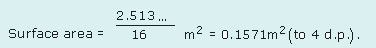Type of slabSurface area/m2
Central slab0.1257Lightest
Inner ring slab0.1885Heaviest
Outer ring slab0.1571

Assuming that the slabs are of equal thickness, and are made of the same material, the weights of the slabs will be proportional to the surface areas. So the results show that the lightest slabs are in the central circle and the heaviest in the inner ring.

## Hint

Try breaking the problem in question 4 down into steps and considering what you know about what you want to find out. You know the radii of the circles, so can find the area of each circle. That should help you to find the area of each ‘ring’ of slabs. Then count how may slabs are in each ring and use that to work out the area of each slab.